28th International Conference on Technology in Collegiate Mathematics2016
¤
March 12, 2016
Mapping Diagrams for Complex Variable Functions
Visualized Dynamically with GeoGebra
1:30 p.m. - 2:00 p.m.

 Part I Mapping Diagrams for Real Functions Complex Arithmetic Part II Complex Functions Part III Calculus for Complex Functions

Martin Flashman
Professor of Mathematics
Humboldt State University

http://flashman.neocities.org/Presentations/MD.ICTCM.CV.3_12_16.html

Abstract: Mapping diagrams provide a valuable tool for visualizing complex variable functions. Using GeoGebra, demonstrated dynamic diagrams will allow users to see important properties of complex functions. Examples include complex arithmetic, key functions, and calculus concepts.
Background and References to other work on Mapping Diagrams for real variables ¤

1.1.Background: Mapping Diagrams for Real Functions
1.1.1 Real Functions. ¤
Understanding Real Functions: Tables, Mapping Diagrams, and Graphs

1.2. Real Linear Functions. ¤
Real Linear functions are the key to understanding calculus.
Linear functions are traditionally expressed by an equation like :
$f(x)= mx + b$.
Mapping diagrams for real linear functions have one simple unifying feature- the focus point, determined by the numbers
$m$ and $b$, denoted here by $[m,b]$
.
Mapping Diagrams and Graphs of Real Linear Functions
Visualizing real linear functions using mapping diagrams and graphs.
This is a Java Applet created using GeoGebra from www.geogebra.org - it looks like you don't have Java installed, please go to www.java.com

Notice how points on the graph pair with arrows and points on the mapping diagram.

2.1 A Review of Complex Numbers and Arithmetic:     $\mathbb{C}$¤
Definition: The "number" $i$:

To solve the equation $x^2 +1 =0$ we create a symbol $i$ and declare that $i^2 = -1$ so $i$ solves the equation  and we write $$i = \sqrt{ -1 }.$$

Definitions: A complex number $z$ is a number that can be expressed in the form $z =a +bi$ where $a$ and $b$ are real numbers.

If
$z =a +bi$ then $a$ is called the real part of $z$ and $b$ (and sometime $bi$) is called the imaginary part of $z$.
Examples: $3 + 2i$ and $-2 - i$.

 2.2 Complex Geometry- The Complex Plane Complex numbers are identified with points in a cartesian plane by having $a +bi$ identified with the point with coordinates $(a,b)$ or with position vectors by identifying $a+bi$ with the vector $$. With this identification i is identified with the point (0,1) and -i is identified with (0,-1). 2.2.1 Complex Number Norm (Magnitude): The norm of z is defined by |z| = |a+bi| = \sqrt{ a^2 +b^2} 2.2.2 Polar Representation of z: ¤ Using trigonometry we have the identification:$$z = |z| \cos( \theta) + |z| \sin(\theta) i = |z| [\cos( \theta) + \sin(\theta) i] = |z| cis( \theta) $$where a = \cos( \theta ), b = \sin(\theta). The angle \theta determined by z can be measured in degrees or radians and restricted to be in a specific interval. For example \theta \in [0,2 \pi) or \theta \in (-\pi, \pi]. Thus the angle can be considered a function of z, called the argument of z: Arg(z) =\theta.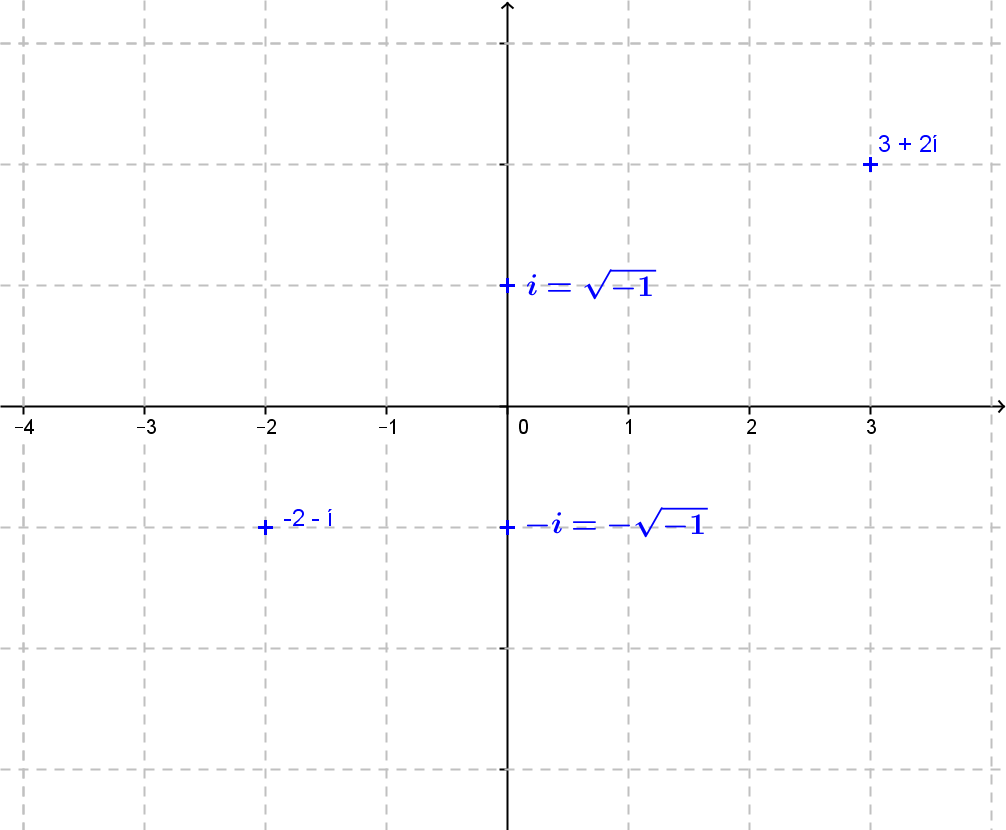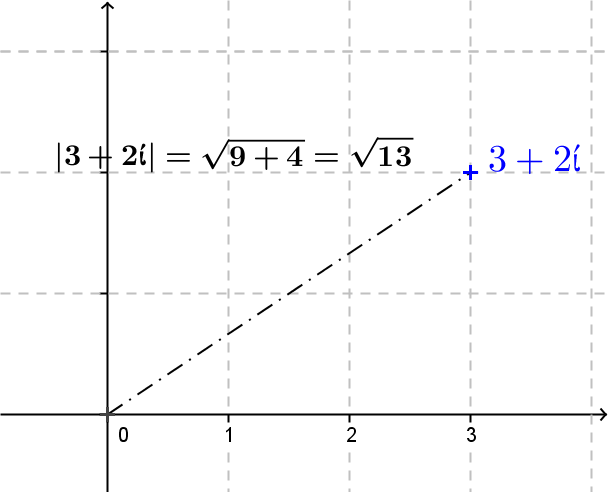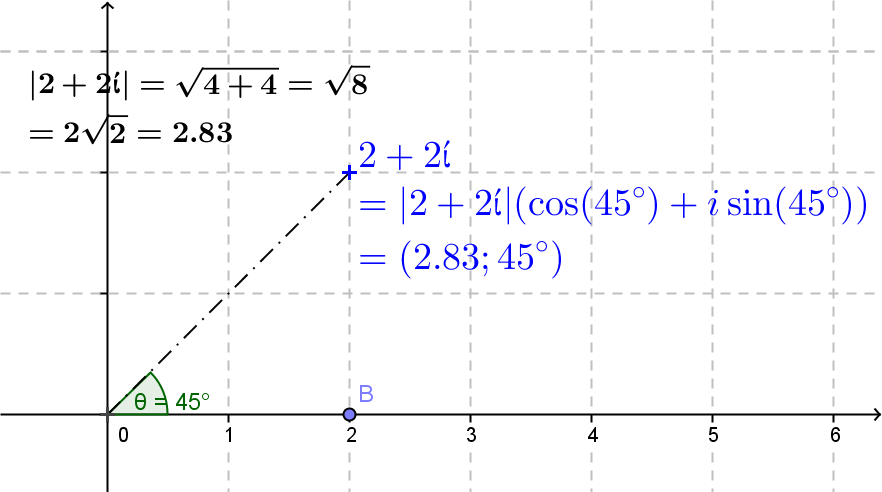2.2.3 Exponential Representation of z: ¤ Consider the Taylor Series for the functions:$$e^x = 1 + x + \frac{x^2}2 +\frac{x^3}{3!} + \frac{x^4}{4!} + \frac{x^5}{5!} + \frac{x^6}{6!} + \frac{x^7}{7!}  ...\cos(x) = 1 - \frac{x^2}2 + \frac{x^4}{4!} - \frac{x^6}{6!} + ...\sin(x) =  x - \frac{x^3}{3!} +  \frac{x^5}{5!}  -  \frac{x^7}{7!}  ...$$Then using \theta in radian measure:$$e^{i\theta}= \cos(\theta) + \sin(\theta)i = cis (\theta)$$and$$z =|z|e^{i \theta}$$. Note: When \theta =\pi this equation demonstrates that e^{\pi i} = \cos(\pi) + sin(\pi)i = -1. 2.3 Complex Arithmetic  2.3.1 Complex Addition: z_1+z_2 ¤ If z_1 = a_1 + b_1i and z_2 = a_2 + b_2i then$$z_1+z_2 =a_1 + a_2 + (b_1+ b_2) i  Example: If $z_1 = 2+3i; z_2= 1 - i$ then  $z_1+z_2= (2+1) + (3-1) i = 3 + 2i$. The addition can be thought of as vector addition        - with separate addition of the real and imaginary parts. Addition can be visualized in the complex plane by using parallelograms.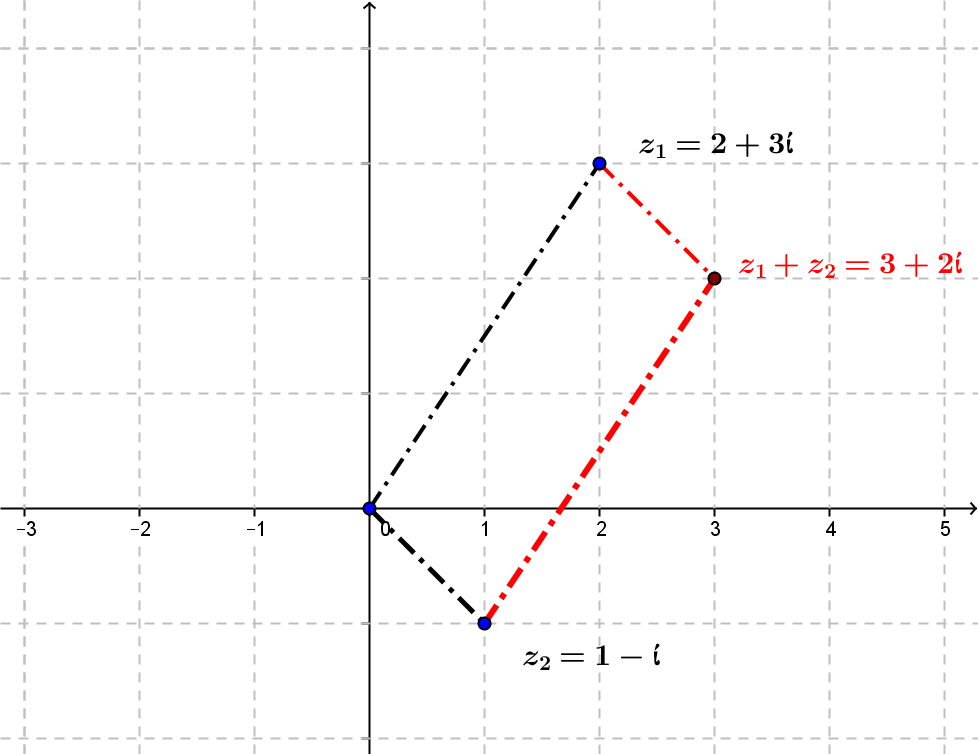2.3.2 Complex Multiplication: $z_1 \cdot z_2$   ¤
 Algebraically:If $z_1 = 2+3i; z_2= 1 - i$ then  $z_1 \cdot z_2= (2+3i) \cdot (1-i) = 2 \cdot 1 - 3\cdot i^2 + 3i \cdot 1 +2 \cdot (-i) = (2+3) +(3- 2)i = 5+i$. This is easier to understand complex multiplication geometrically using the polar  or the exponential representation (in radian measure): $z_1=|z_1| [\cos( \theta_1) + \sin(\theta_1) i] = |z_1|e^{i\theta_1} ;\ z_2=\cdot |z_1| [\cos( \theta_2) + \sin(\theta_2) i] = |z_2|e^{i\theta_2}$ $z_1 \cdot z_2= |z_1| [\cos( \theta_1) + \sin(\theta_1) i] \cdot |z_1| [\cos( \theta_2) + \sin(\theta_2) i]$        $= |z_1|\cdot |z_1| [\cos( \theta_1) + \sin(\theta_1) i] \cdot [\cos( \theta_2) + \sin(\theta_2) i]$        $= |z_1|\cdot |z_1| [\cos( \theta_1)\cos( \theta_2) - \sin(\theta_1)\sin(\theta_2) + (\sin(\theta_1)\cos( \theta_2) + \sin(\theta_2) \cos(\theta_1) )i]$        $=|z_1|\cdot |z_1| [\cos( \theta_1 + \theta_2) + \sin(\theta_1 +\theta_2) i]$        $=|z_1|\cdot |z_1| cis (\theta_1 +\theta_2)$ or more simply using $\theta$ in radian measure: $z_1 \cdot z_2= |z_1|e^{i\theta_1}\cdot |z_2|e^{i\theta_2}$         $=|z_1|\cdot |z_1| e^{(\theta_1 +\theta_2)i}$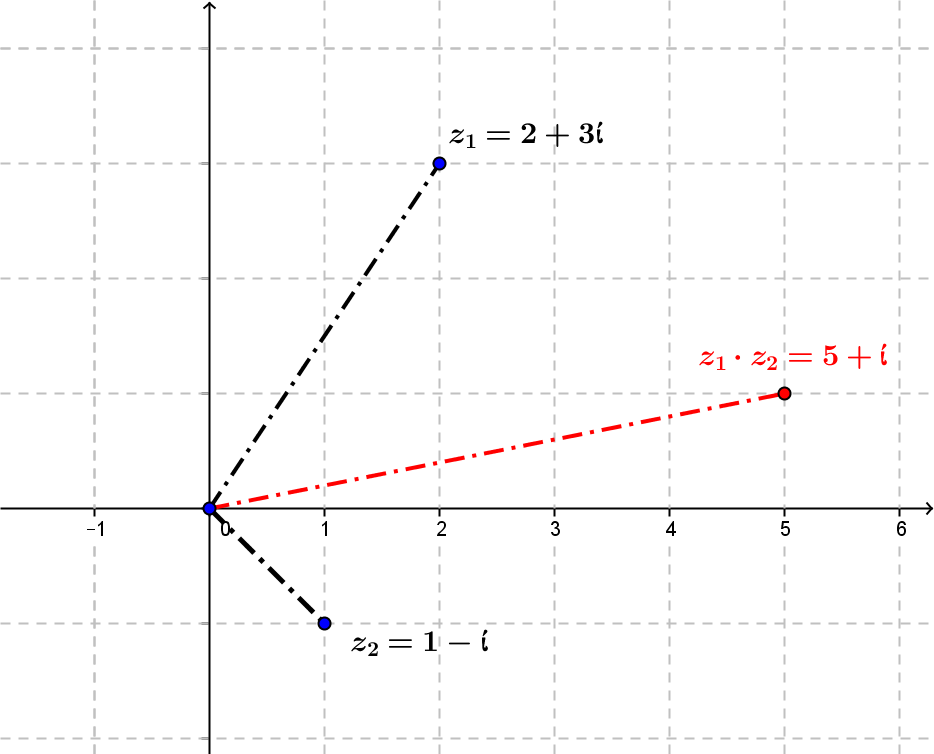Example: If $z_1 = \sqrt 2\ cis(45°); z_2= 2\ cis(30°)$ then  $z_1\cdot z_2= 2\sqrt 2\ cis (75 °)$.¤

2.3.3 Complex Inverses: $\frac1z, z \ne 0$ ¤
 Example: Express $\frac 1{2+3i}$ in the standard form of $a + bi$. Solution: $\frac 1{2+i} =\frac 1{2+i} \cdot \frac {2-i}{2-i} = \frac{2-i}{4+1} = \frac 2{5} - \frac 3{5}i$. Fact: If $a^2+b^2 \ne 0$ then  $\frac1{a+bi} = \frac a{a^2 + b^2} - \frac b{a^2 + b^2}i$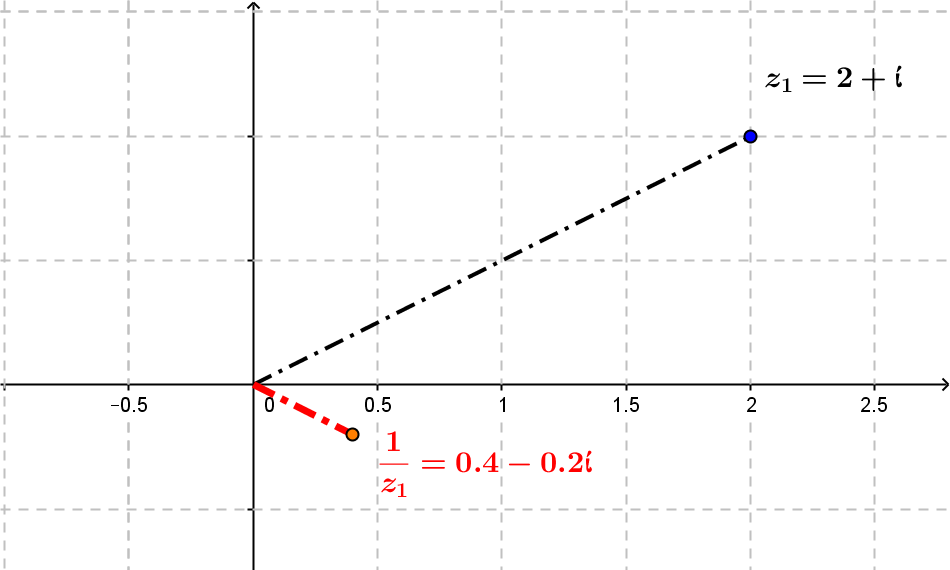2.3.4  Geometry for $\frac1z, z \ne 0$
Geometric understanding of $\frac1z, z \ne 0$ comes best by using the polar or exponential representation.

That is, if $z=|z|\ cis(\theta) = |z|e^{i\theta}$ then $\frac1z = \frac1{|z|} \ cis(-\theta) = \frac1{|z|} e^{-i\theta}$